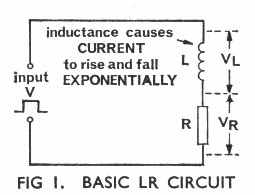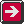Main Radar Home Radar theory Home AP3302 Pt3 Contents AP3302 Pt3 Section 2Contents AP 3302 Pt. 3 Section 2 CHAPTER 3 Square Waves applied to LR and LC circuits Introduction We have seen in Part 1 of these notes that a coil of wire has the property of inductance which has the effect of opposing any change in the value of current flowing through the coil. It does this by producing a self-induced voltage, or back e.m.f, which is of opposite polarity to the appliedvoltage. Circuits which contain inductance L and resistance R are known as LR circuits (Fig 1). When we apply a square wave of voltage to a LR circuit the resultant waveforms of VL and VR. are similar to the waveforms of VR. and VC we obtain from CR circuits. LR circuits are not often used deliberately in practice in the way that CR circuits are used, because a capacitor is normally needed to 'block' the d.c. component of the resultant waveform. However, every inductor has resistance as well as inductance and so acts as a LR circuit, Allowance must there-fore be made for this in the design of radar circuits because of the effect which the application of a square wave to a LR circuit has. Let us see something of this effect. Effect of Applying a Square Wave to a LR Circuit In Fig 2a we have a LR circuit and a simple 'square wave generator', i.e. the battery and the switch. With the switch in the OFF position the voltage V applied to the LR circuit is zero, VL is zero and so is VR. a. A to B. When we switch ON, the current cannot rise to its maximum value instantaneously because the back e.m.f. opposes any rise in the current. Initially therefore there is no current in the circuit and the voltage VR. must be zero (VR= I x R). The whole of the applied voltage V thus appears across L and VL rises immediately to the voltage V of the supply, in this case +100V; VR. at this instant is zero (Fig 2b). b. B to C. Subsequently the current begins to rise and will continue to rise exponentially on a time constant of L/R seconds until, if given time, it reaches its maximum value. Thus VR. rises exponentially and VL falls at the same rate (at all times VR. + VL = V). After a time equal to 5L/R seconds a steady state is reached where VR. = 100V and VL = zero. c. C to D. If now we switch OFF, the applied voltage V falls immediately from +100V to zero. However, the current in the circuit cannot change instantaneously, because of the effect of the inductance, and the voltage VR. will remain initially at +100V. Since at all times V = VR. + VL and the applied voltage V is zero, we have at this instant: 0 = VR. + VL \ VL = -VR. = -100V. Thus at the instant of switching OFF, the voltage VL across L falls immediately to -100V. d. D to E. Subsequently the current in the circuit falls exponentially on a time constant of L/R seconds, causing VR. to fall exponentially from +100V towards zero and VL to rise exponentially from -100V towards zero (Fig 2b). Both voltages are zero after 5L/R seconds.Previous page To top of this page Next Page

Constructed by Dick Barrett
Email: